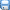## 2015版七年级数学上册提技能+题组训练 3.3.1《一元一次方程的解法》（第1课时） 湘教版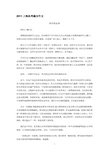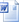资料类别:  数学/同步 所属版本:  浙教版 所属地区:  全国 上传时间:  2015/11/5 下载次数:  21 次 资料类型: 上传人:  hYZe****@yahoo.com.cn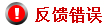### 资料概述与简介

3.3.1 一元一次方程的解法（第1课时） 提技能·题组训练 用移项解一元一次方程 1.下列方程的变形中,是移项的是　(　　) A.由3=x,得x=3 B.由6x=3+5x,得6x=5x+3 C.由2x=-1,得x=- D.由2x-3=x+5,得2x-x=5+3 【解析】选D.选项A中等号左右两边交换,但是没有变号,不是移项;选项B中等号右边的5x与3交换位置不是移项;选项C中是等号两边同时除以2,不是移项;选项D中等号右边的x变为-x移到等号左边,等号左边的-3变为3移到等号右边,是移项. 【易错提醒】移项是指把等式一边的某项变号后移到另一边,而在等号的同一侧变动某项的位置不是移项,也不需要改变符号. 2.解方程6x+1=-4,移项正确的是　(　　) A.6x=4-1 B.-6x=-4-1 C.6x=1-4 D.6x=-4-1 【解析】选D.选项A,B的错误是没移动的项符号发生了改变;选项C中的1从左移到右,而符号没改变. 3.(2014·厦门汀溪中学质检)方程2x-4=0的解是　　　　　　　. 【解析】移项,得2x=4, 两边都除以2,得x=2. 答案:x=2 4.3x与1+2x互为相反数,则x=　　　　　. 【解析】由题意得3x+1+2x=0, 移项,得3x+2x=-1,合并同类项,得5x=-1, 两边都除以5,得x=-. 答案:- 5.已知当x=2,y=1时,代数式kx-y的值是3,那么k的值是　　　　. 【解析】由题意,得2k-1=3, 移项,得2k=3+1, 合并同类项,得2k=4, 两边都除以2,得k=2. 答案:2 6.解方程:4x+5-3x=3-2x. 【解析】移项,得4x-3x+2x=3-5. 合并同类项,得3x=-2. 两边都除以3,得x=-. 解带括号的一元一次方程 1.解方程3-(x+6)=-5(x-1)时,去括号正确的是(　　) A.3-x+6=-5x+5 B.3-x-6=-5x+5 C.3-x+6=-5x-5 D.3-x-6=-5x+1 【解析】选B.选项A,C均出现了符号错误;选项D出现了漏乘. 2.方程-3(x+1)=9的解为　(　　) A.x=-2　 B.x=-4　 C.x=2　　 D.x=3 【解析】选B.去括号,得-3x-3=9,移项,得-3x=12,两边都除以-3,得x=-4. 【一题多解】选B.把(x+1)看作一个整体,方程的两边都除以-3,得x+1=-3,移项,得x=-4. 3.当x=　　　　时,5(x-2)的值等于8. 【解析】根据题意得5(x-2)=8,去括号,得5x-10=8,移项,得5x=18,两边都除以5,得x=. 答案: 4.(2014·江苏外国语学校质检)若方程4(a-x)-4(x+1)=60的解是x=-1,则a为　　　　. 【解题指南】 【解析】把x=-1代入得4(a+1)=60,去括号,得4a+4=60,移项,得4a=56,两边都除以4,得a=14. 答案:14 5.若a,b,c,d为有理数,现规定一种新运算:=ad-bc,那么当=18时,x=　　　　. 【解析】由新运算得2×5-4(1-x)=18. 去括号,得10-4+4x=18.移项,得4x=18-10+4. 合并同类项,得4x=12.两边同除以4,得x=3. 答案:3 6.小明星期天从家里出发骑自行车去书店买书,去时顺风用了15min,回来时逆风用了20min.已知小明骑自行车的速度不变,为280m/min,则风速为　　m/min. 【解析】设风速为xm/min,则 15(x+280)=20(280-x),解得x=40. 答案:40 7.解方程:5(3-x)-12(5-2x)=-17. 【解析】去括号,得15-5x-60+24x=-17, 移项,得-5x+24x=-17-15+60, 合并同类项,得19x=28,两边同除以19,得x=. 8.当x为何值时,式子3(x-2)和4(x+3)-4相等. 【解析】根据题意,得3(x-2)=4(x+3)-4,去括号,得3x-6=4x+12-4,移项,得3x-4x=12-4+6,合并同类项,得-x=14,两边都除以-1,得x=-14. 答:当x=-14时,式子3(x-2)和4(x+3)-4相等. 【变式训练】如果2(x+3)的值与3(1-x)的值互为相反数,那么x=　　　　. 【解析】因为2(x+3)与3(1-x)互为相反数, 所以2(x+3)+3(1-x)=0,去括号,得2x+6+3-3x=0,移项得,2x-3x=-6-3,合并同类项得-x=-9, 两边都除以-1,得x=9. 答案:9 【错在哪？】作业错例 课堂实拍 解方程:3(x-7)-2(9-2x)=18. (1)找错:从第_______步开始出现错误. (2)纠错: ______________________________ ________________________________________ ________________________________________ 答案: (1)① (2)去括号,得3x-21-18+4x=18, 移项,得3x+4x=18+21+18, 合并同类项,得7x=57, 两边都除以7，得x= 初中学习网，资料共分享！我们负责传递知识！www.czxxw.com

## 更多>>其他相关资源### 资料ID：

/ /

…下载本资料需要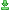下载次